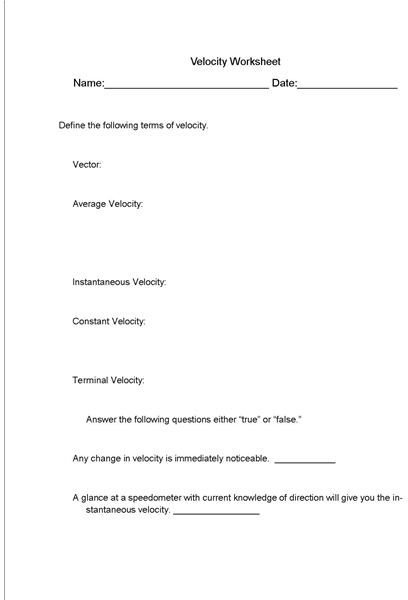What is Velocity? All is Revealed in This Science Lesson Plan

What is Velocity?

Explain velocity in motion to your students and then have them complete the worksheet included in the final section below and review the velocity study guide that accompanies this lesson plan. When used together, this complete velocity package should assist in developing a student’s understand of velocity, its terms and how it is used.

Explain the following to your students.

Velocity is a vector quantity. This means that velocity expresses both speed and direction. Therefore velocity can also be used to find acceleration due to the fact that acceleration is determined by changes in velocity.

Velocity Board Work

Explain the following information to students and ask them to think of how velocity is useful in the following situations. Discuss possible issues that could result from a lack of knowledge of velocity in these situations.

• Three airplanes are coming in for landing. Given that they are all going 200 mph, how would velocity be useful in determining where and when they could each land?
• The wind is blowing at 12 mph. A group of skydivers are attempting to jump from a plane. How would the velocity of the wind or the airplane be useful in determining where the skydivers should jump from?
• If the police are chasing a runaway car, why is the velocity of the car important information to have?
• Consider how a skydiver spreads their arms and legs as they are falling. What might this do to the air resistance as far as terminal velocity is concerned?
• Why is speed a vital part of expressing velocity?

Discuss with your students how velocity is used in everyday situations and how it is useful to us. Use comparisons like skateboarding and biking to help students understand how velocity is used. Review the worksheet and velocity study guide with students to help them understand velocity, its terms and the formulas used to determine velocity.

Velocity WorksheetThis post is part of the series: Motion in Physical Science

This series on motion in physical science comes complete with worksheets and study guides for motion, speed, velocity and acceleration.